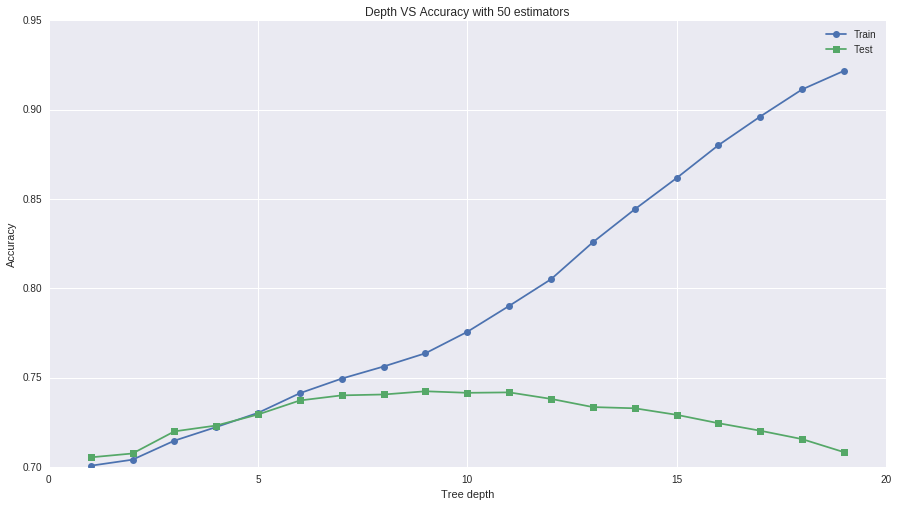# samucoder

Samuel M.H. 's technological blog

Notebook

# Running a Random Forest

Author: Samuel M.H. <samuel.mh@gmail.com> Date: 04-03-2016

## Instructions

The second assignment deals with Random Forests. Random forests are predictive models that allow for a data driven exploration of many explanatory variables in predicting a response or target variable. Random forests provide importance scores for each explanatory variable and also allow you to evaluate any increases in correct classification with the growing of smaller and larger number of trees.

### Run a Random Forest.

You will need to perform a random forest analysis to evaluate the importance of a series of explanatory variables in predicting a binary, categorical response variable.

## What to Submit

Following completion of the steps described above, create a blog entry where you submit syntax used to run a Random Forest (copied and pasted from your program) along with corresponding output and a few sentences of interpretation. Please note that your reviewers should NOT be required to download any files in order to complete the review.

## Intro

This week I will look for nonlinear relationships in order to predict if a person works 35 or more hours a week. This will be done with a random forest model. The baseline for the accuracy is 0.73214948645 which was obtained in the previous assignment with a classification tree of depth 4.

## Dataset

### Variables

• Response:

• WORK35 -> S1Q7A1: present situation includes working full time (35+ hours a week). Categorical yes/no.
• Explanatory:

• AGE -> AGE: age (years).
• S1Q24LB -> WEIGHT: weight (pounds).
• NUMPERS -> HOUSE_PEOPLE: number of persons in household.
• ETHRACE2A -> RACE: imputed race/ethnicity (5 groups, reference group=1,white).
• SEX -> MALE: gender (2 groups).
• S10Q1A63 -> CHANGE_MIND: change mind about things depending on people you're with or what read or saw on tv (2 groups).
In :
%pylab inline

import numpy as np
import pandas as pd

from sklearn.cross_validation import train_test_split
from sklearn.ensemble import RandomForestClassifier, ExtraTreesClassifier
from sklearn.metrics import classification_report
import sklearn.metrics

#Visualization
import seaborn as sns

pylab.rcParams['figure.figsize'] = (15, 8)

Populating the interactive namespace from numpy and matplotlib



## Data

In :
# Load data

In :
# Custom dataframe
df = pd.DataFrame()

# Response variable
df['WORK35'] = data['S1Q7A1'].replace(' ',np.NaN).replace('2','0').astype(float)

# Explanatory variables
df['AGE'] = data['AGE'].replace(' ',np.NaN).replace('98',np.NaN).astype(float)
df['WEIGHT'] = data['S1Q24LB'].replace(' ',np.NaN).replace('999',np.NaN).astype(float)
df['HOUSE_PEOPLE'] = data['NUMPERS'].replace(' ',np.NaN).astype(float)
df['RACE'] = data['ETHRACE2A'].replace(' ',np.NaN).astype('category')
df['MALE'] = data['SEX'].replace(' ',np.NaN).replace('2','0').astype('category')
df['CHANGE_MIND'] = data['S10Q1A63'].replace(' ',np.NaN).replace('9',np.NaN).replace('2','0').astype('
category')

df = df.dropna()


Note: data summaries, counts and descriptions can be seen in the previous assingment.

### Split: train, test

In :
TARGET = 'WORK35'
PREDICTORS = list(df.columns)
PREDICTORS.remove(TARGET)

df_target = df[TARGET]
df_predictors = df[PREDICTORS]

train_target, test_target, train_predictors, test_predictors = train_test_split(df_target, df_predictors, test_size=0.4, random_state=42)

print('Samples train: {0}'.format(len(train_target)))
print('Samples test:  {0}'.format(len(test_target)))

Samples train: 24242
Samples test:  16162



## Model

In :
model1 = RandomForestClassifier(n_estimators=25)
model1.fit(train_predictors, train_target)

Out:
RandomForestClassifier(bootstrap=True, class_weight=None, criterion='gini',
max_depth=None, max_features='auto', max_leaf_nodes=None,
min_samples_leaf=1, min_samples_split=2,
min_weight_fraction_leaf=0.0, n_estimators=25, n_jobs=1,
oob_score=False, random_state=None, verbose=0,
warm_start=False)


### Metrics

In :
predictions=model1.predict(test_predictors)

print('Confusion matrix [[TP,FP],[FN, TN]]')
print(sklearn.metrics.confusion_matrix(test_target,predictions))

print('\nAccuracy')
print(sklearn.metrics.accuracy_score(test_target, predictions))

Confusion matrix [[TP,FP],[FN, TN]]
[[5098 2640]
[2317 6107]]

Accuracy
0.693292909293



The accuracy of this model is not as good as the baseline. Maybe the model is overfit due to the depth of the random forests. Lets test this hypothesis.

## Improving the model

I am going to generate several models limiting the depth of the random trees from 1 to 20 nodes.

In :
#Results
acc_train = {} #Accuracy on training dataset
acc_test = {} #Acc on testing dataset

for depth in xrange(1,20):
modeln = RandomForestClassifier(n_estimators=50, n_jobs=4, max_depth=depth)
modeln.fit(train_predictors, train_target)
acc_train[depth] = modeln.score(train_predictors,train_target)
predictions=modeln.predict(test_predictors)
acc_test[depth] = sklearn.metrics.accuracy_score(test_target, predictions)

In :
plt.xlabel('Tree depth')
plt.ylabel('Accuracy')
plt.title('Depth VS Accuracy with 50 estimators')
plt.plot(acc_train.keys(),acc_train.values(),  marker="o", label="Train")
plt.plot(acc_test.keys(),acc_test.values(),  marker="s", label="Test")
plt.legend()
plt.show()Measuring the accuracy of the model with the testing dataset, it is possible to see the accuracy increases with the tree depth reaching its maximum value at 10 and decreasing monotonically from this point. If this curve (green) is compared with the one obtained from the training dataset (blue), it is possible to see the original model was overfitted.

### Metrics

In :
model10 = RandomForestClassifier(n_estimators=50, n_jobs=4, max_depth=10)
model10.fit(train_predictors, train_target)

predictions=model10.predict(test_predictors)

print('Confusion matrix [[TP,FP],[FN, TN]]')
print(sklearn.metrics.confusion_matrix(test_target,predictions))

print('\nAccuracy')
print(sklearn.metrics.accuracy_score(test_target, predictions))

Confusion matrix [[TP,FP],[FN, TN]]
[[4849 2889]
[1289 7135]]

Accuracy
0.741492389556



The accuracy is improved, it's even better than the baseline but it is possible to see there is a problem with the false positives.

### Features importance

In :
#Chosen model
sorted(zip(PREDICTORS,model10.feature_importances_),key=lambda x:x,reverse=True)

Out:
[('AGE', 0.70263154725375743),
('WEIGHT', 0.10337365584777715),
('MALE', 0.086455602023945227),
('HOUSE_PEOPLE', 0.071275426739257131),
('RACE', 0.027183899676646889),
('CHANGE_MIND', 0.0090798684586160285)]


This features importance are provided by the model but they are not used in the course. In the single tree model, the age is the most important predictor followed by the sex. Lets try with other model to properly interpret this measure.

## Extra tree classifier

In :
#With extra trees classifier
model_feat = ExtraTreesClassifier(n_estimators=50, n_jobs=4, max_depth=10)
model_feat.fit(train_predictors,train_target)

Out:
ExtraTreesClassifier(bootstrap=False, class_weight=None, criterion='gini',
max_depth=10, max_features='auto', max_leaf_nodes=None,
min_samples_leaf=1, min_samples_split=2,
min_weight_fraction_leaf=0.0, n_estimators=50, n_jobs=4,
oob_score=False, random_state=None, verbose=0, warm_start=False)


### Metrics

In :
predictions=model_feat.predict(test_predictors)

print('Confusion matrix [[TP,FP],[FN, TN]]')
print(sklearn.metrics.confusion_matrix(test_target,predictions))

print('\nAccuracy')
print(sklearn.metrics.accuracy_score(test_target, predictions))

Confusion matrix [[TP,FP],[FN, TN]]
[[4863 2875]
[1414 7010]]

Accuracy
0.73462442767



### Features importance

In :
sorted(zip(PREDICTORS,model_feat.feature_importances_),key=lambda x:x,reverse=True)

Out:
[('AGE', 0.72949165584031528),
('MALE', 0.14741349854526331),
('HOUSE_PEOPLE', 0.062456138576466778),
('WEIGHT', 0.032239073237816517),
('RACE', 0.021686475674321783),
('CHANGE_MIND', 0.0067131581258164427)]


## Conclusion

I have improved the base accuracy from the single tree model. No tree can be interpreted but the feature importance have been calculated and the age is the most relevant predictor followed by the sex, which corresponds with the conclusion extracted from the single tree model. The advantage of this method is that its generalization power is far superior because the variance reduction due to the processes of generating random trees.

Although the good results it seems I've reached the maximum prediction accuracy a machine learning model can provide, in order to predict if a person has a full time job, I should add more predictors, but this assignment is focused on testing and evaluating algorithms, not on predicting anything.